#### You may also like### Double Digit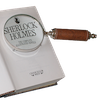### Number Detective

Follow the clues to find the mystery number.### Six Is the Sum

What do the digits in the number fifteen add up to? How many other numbers have digits with the same total but no zeros?

# More Less Is More

##### Age 7 to 14Challenge Level

Sum-sum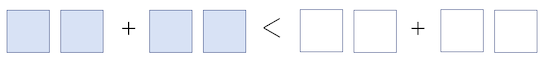Gerard from Frederick Irwin Anglican School in Australia inserted numbers that make the inequality true:

32+36 is less than 65+26 this is because 32+36=68 while 65+26=91

Gerard's score is 68.

Leticia and Amelie from Halstead Prep School and Rishaan, Swarnim and Eshaan from Ganit Kreeda in India worked out how to get the highest score using the numbers 1 to 8. This is Leticia's work:

I got the four highest numbers 5, 6, 7 and 8. I then arranged them do that I had 5 and 8 on the left hand side and 6 and 7 on the right hand side. I then had the other four numbers and I then figured out that I could do 1+3=4 and 4+2=6 and you can’t have 3+2 and 1+4 because these both add up to five so they are equal. You can’t do 2+1 on the left hand side because that is not the highest combination. So I then did 51 and 83 on the left and 64 and 72. If you did this the other way round the inequality would be incorrect. Then I added 51 and 83 and then I got 134 which is the highest score.

Gowri from Ganit Kreeda explained how to maximise your score given any 8 numbers: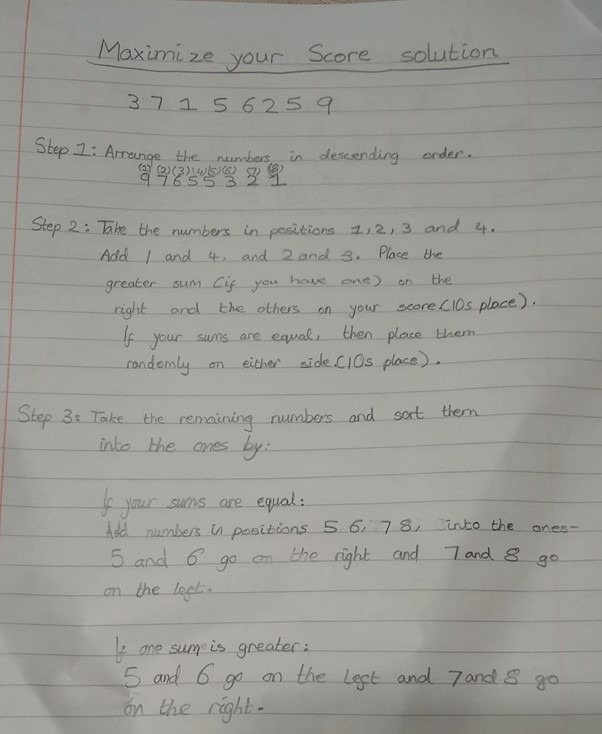Take-take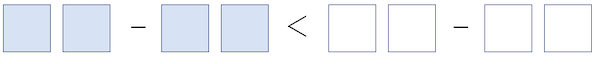Gerard inserted numbers that make the inequality true:

54-56 is less than 44-26 as 54-56= -2

Gerard's numbers give a negative score of -2.

Eshaan, Rishaan, Samaira, Gowri, Vibha, Viha, Vansh, Vraj, Arya, Swarnim, Rudraraj and Renah from Ganit Kreeda worked together to find the best score possible using the numbers 1 to 8:

Swarnim, Vansh and Eshaan had used the strategy of greatest number – smallest number to get the maximum difference.
Rishaan tried the numbers at tens place such as the difference will be maximum and equal for both the sides.
As 8-2=7-1 = 6
He used 8_ - 2_ < 7 _ - 1_
The kids then used the remaining digits at ones place. Again, they used the same strategy as used in the first challenge. They tried to get the difference of 1.
85 – 24 < 76 – 13 or 61 < 63
The Highest Score is 61.

Amelie used very similar reasoning, expressed in a different way: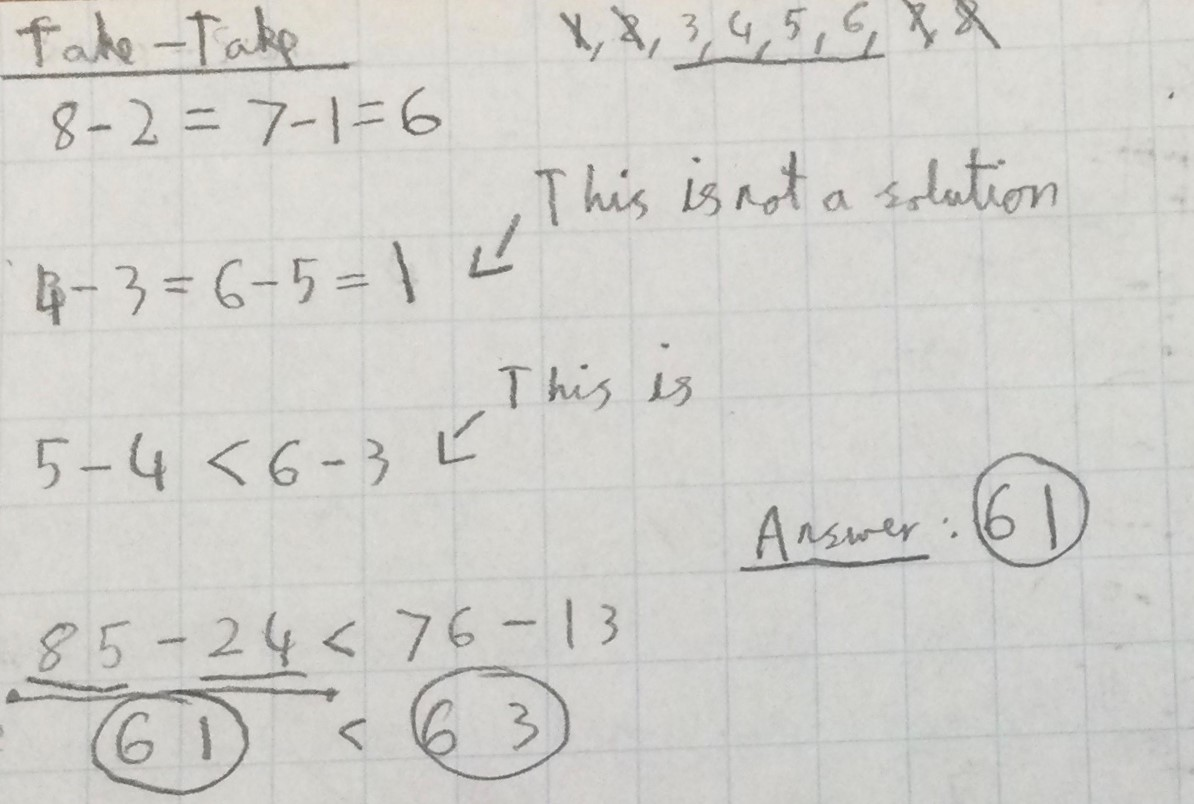Take-sum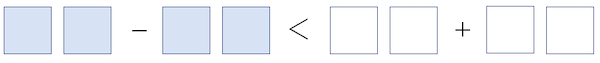Gerard inserted numbers that make the inequality true:

31-45 is a negative while 34+14 is positive

Again, Gerard's score is negative (this time -14).

Amelie combined the numbers 1 to 8 to create a higher score: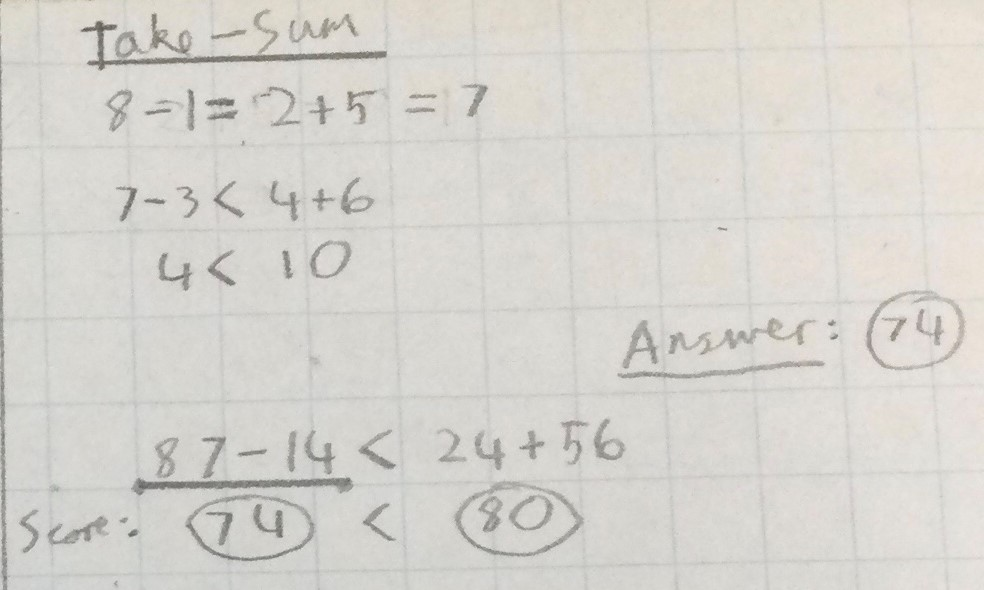Note that Amelie probably meant 87-13 rather than 87-14.

The students from Ganit Kreeda managed to get a slightly higher score:

87-12 will give the maximum score we can get on the left side which is 75 and now to get a number bigger than this on the right side we can use the leftover numbers.

87 - 12 < 35 + 46

Sum-take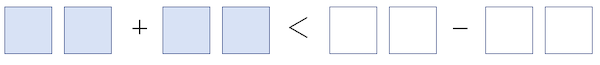Gerard inserted numbers that make the inequality almost true:

12+11 is less than 46-23

In fact, now both sides are equal to 23, so this is not actually true!

Amelie used the numbers 1 to 8 to create a high score: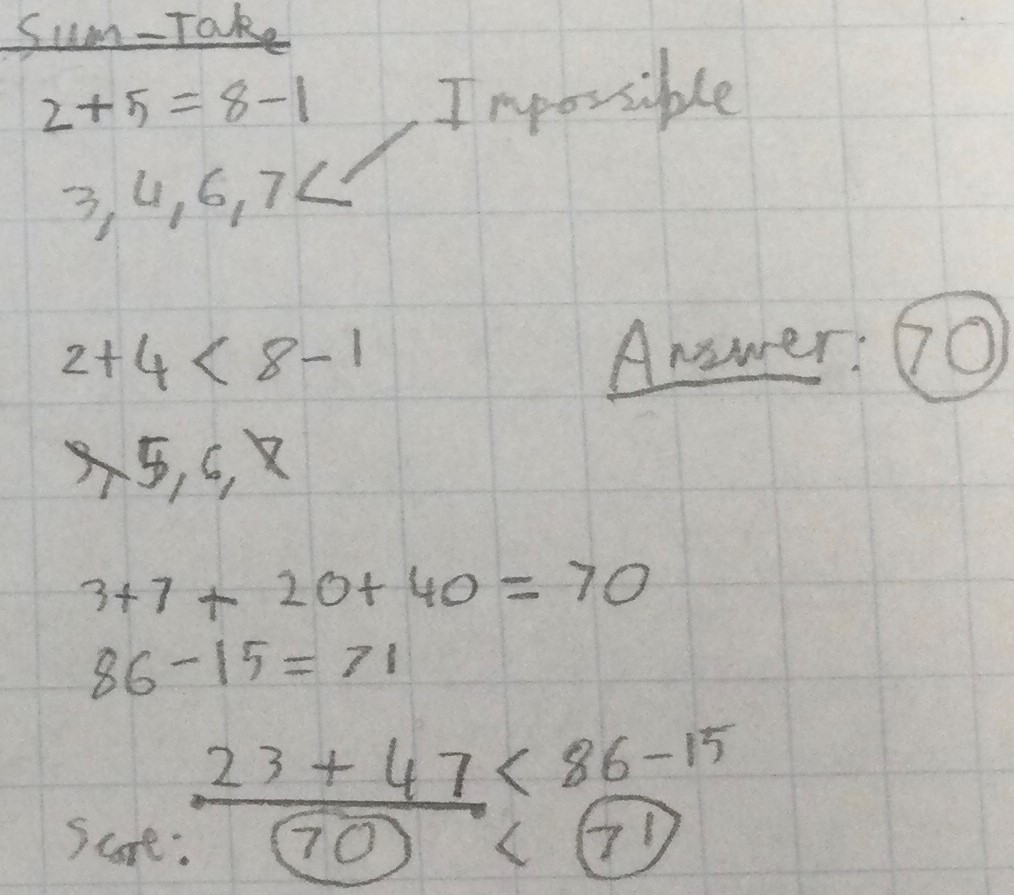The students from Ganit Kreeda also got a score of 71:

First, we tried to use maximum difference which is 75 on the right side.

But then using remaining digits, the smallest possible addition was 35+46 which is 81, and 81>75.

So, we tried to make right side 74 by changing 12 to 13 as 87-13.

With remaining digits we got 26+45=71 and 87–13=74 OR 46+25<87-13

The Highest Score is 71.

It is actually possible to get a score of 72. Can you see how?